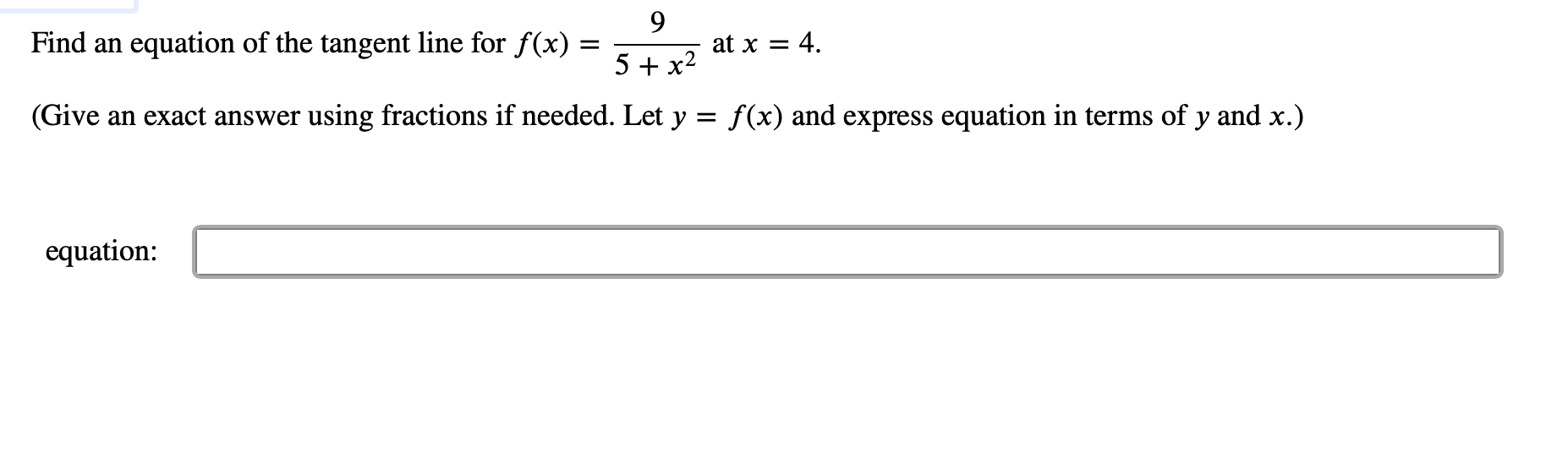# 9 at x 4 Find an equation of the tangent line for f(x) 5 x2 f(x) and express equation in terms of y and x.) using fractions if needed. Let y (Give an exact answer equation

Questionhelp_outlineImage Transcriptionclose9 at x 4 Find an equation of the tangent line for f(x) 5 x2 f(x) and express equation in terms of y and x.) using fractions if needed. Let y (Give an exact answer equation fullscreen

### Want to see this answer and more?

Experts are waiting 24/7 to provide step-by-step solutions in as fast as 30 minutes!*

*Response times vary by subject and question complexity. Median response time is 34 minutes and may be longer for new subjects.
Tagged in
MathCalculus

### Derivative